Next: Image Restoration Up: Image Manipulations Previous: Background Estimates

Transformations

Depending on the further reductions the data may be transformed into the coordinate system which is most relevant for the physical interpretation. This will typically be used when certain characteristics of the data will appear as a linear relation in the new coordinates. These transformations involve non-linear rebinning as discussed in Section 2.2.3. To conserve flux in the new system, the pixel values must be corrected by the Jacobian determinantin Equation 2.15.

For spectra a transformation from wavelength to frequency is used to identify spectral regions which follow a power law. This transformation is given by(2.21)

where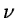is the frequency and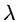is wavelength. The intensities I are translated to a logarithmic scale (e.g. magnitudes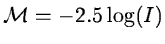) so that a power law spectrum appears linear.

In the classification of galaxies, ellipticals can be distinguished on their radial surface brightness profile which can be approximated by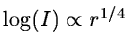. This gives the transformation formula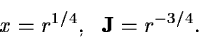(2.22)

Since the intensity profile only should be resampled, the flux correction is not applied in this case. A logarithmic scale is also used here to achieve a linear relation. An example is given in Figure 2.9.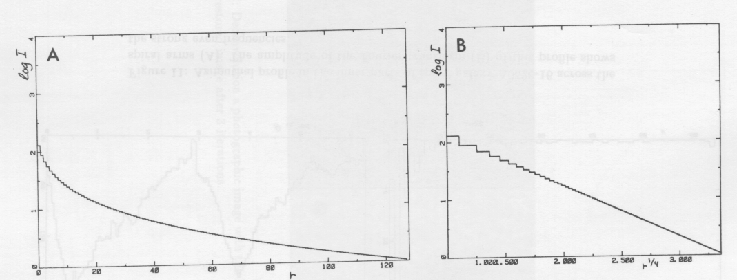Whereas the transforms mentioned above only perform a non-linear rebinning of the data, the Fourier transform converts a spatial image into the frequency domain. This transform has two main applications namely analysis of periodic phenomena and convolution due to its special properties. The transform can be given as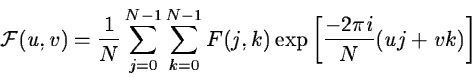(2.23)

where (u,v) are the spatial frequencies and i denotes the imaginary part. The corresponding inverse transform is then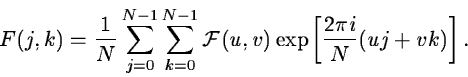(2.24)

Special numeric techniques, called Fast Fourier Transforms or FFT, can significantly decrease the time needed to compute these transforms. They are optimized for images with a size equal to a power of 2 (see e.g. Hunt 1969) but can also be used in other cases.

To analysis the periodic occurrence of features in time series, spectra, or images the amplitude of the Fourier transform or the power spectrum is used. The power spectrumof the function F is given by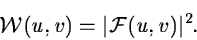(2.25)

Peaks in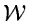indicate the presence of specific frequencies while the continuum originates from a combination of objects and noise. Since the Fourier transform assumes the image to occur periodically, discontinuities at the edges of the image will produce artificial contributions to the power spectrum. Thus, care should be taken to remove systematic background variations to avoid this happening. As an example of using Fourier transforms to extract information from a frame, an azimuthal intensity profile of the spiral galaxy A0526-16 is shown in Figure 2.10.The radius was chosen so that the profile intersects the spiral pattern in the inner parts of the galaxy. In the amplitude plot of the Fourier transform, it can be seen that the spiral pattern mainly contains even frequency components. The 4th and 6thharmonic indicates that the wave is asymmetric due to non-linear effects in the spiral density wave.

It is possible to use the transformation for convolutions because this operation corresponds to a multiplication in the frequency domain :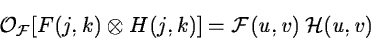(2.26)

where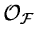anddenote the Fourier transform and convolution operators, respectively. Especially when the convolution matrix is large it is more efficient to perform the operation in frequency than in spatial domain.Next: Image Restoration Up: Image Manipulations Previous: Background Estimates
Petra Nass
1999-06-15